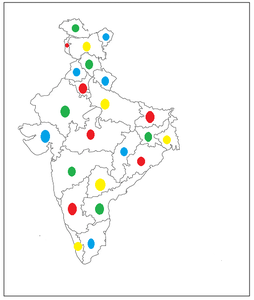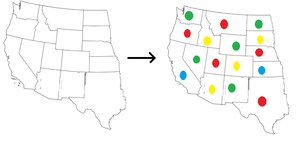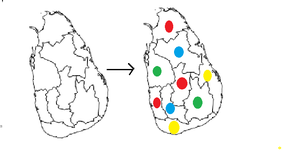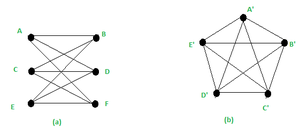# Four Color Theorem and Kuratowski’s Theorem in Discrete Mathematics

• Last Updated : 16 Jul, 2021

Planar Graph :
If a graph can be drawn on the plane without crossing, it is said to be planar.
Coloring of a simple graph is the assignment of color to each vertex of the graph so that no two adjacent vertices are assigned the same color.

Bi-Partite Graphs :
A bipartite graph, also known as a bi-graph, is a set of graph vertices that has been dissected into two distinct sets, with no graph vertices in the same set being adjacent. A bipartite graph is a k-partite graph with k=2 as a particular instance.

Chromatic Number :
The minimum number of colors needed to paint a graph G is called the chromatic number of G & is denoted by – μ (G)

An assignment of colors to the regions of a map such that adjacent regions have different colors.
A map ‘M’ is n – colorable if there exists a coloring of M which uses ‘n’ colors.

Four Color Theorem :
In 1852, Francis Guthrie, a student of Augustus De Morgan, a notable British mathematician and logician, proposed the 4-color problem. He defined the problem in terms of maps that meet specific requirements, such as not having any holes and connecting every region (e.g. country or state) so that no region exists in two or more non-contiguous sections.
Guthrie asserted that with such maps, no more than four colors would be required to color the map so that no two adjacent parts were the same color.
If the regions of a Map M are colored so that adjacent regions are different , then no more than 4 colors are required.
Every planar graph is 4-colorable (Vertex Coloring) but when a triangle is a graph or sub-graph we need only 3 colors .

Mathematicians had been attempting for years to come up with a sophisticated proof (of four color theorem) along the lines of the Six Color Theorem or the Five Color Theorem, and using the brute force method almost appeared like hacking the process.
Every planar graph can be colored in four different ways.
Vertices and edges are found in graphs. We want adjacent vertices/ regions to be of different colors.

How to color ?
Take any map and divide it into a set of connected regions : R1,R2 … Rn with continuous boundaries.
There must be some way to assign each region Ri  -> in the set {R, G, B, Y}  , such that if two regions Ri and  Rj are “touching” (i.e. they share some nonzero length of boundary between them), they must receive different colors.
Example –
1.. The four-color map is shown below :A planar map

Here, as you can see, every region that touches another region has a different color than the touching one & we required a total of a maximum of four colors to color this map – Red, Green, Blue & yellow.

2. The transformation of an uncolored  Map G into a colored Map is  shown below –Map G

Here you can see that every region that touches another region has a different color than the touching one & we required a total of maximum four colors to color this map – Red, Green, Blue & yellow.

3. The transformation of an uncolored  Map H into a colored Map is  shown below –Map H

Here also you can see that every region that touches another region has a different color than the touching one & we required a total of a maximum of four colors to color this map – Red, Green, Blue & yellow.

Kuratowski’s Theorem :
Kuratowski established the theorem establishing a necessary and sufficient condition for planarity in 1930. The theorem states that –

```"If G is non planar if and only if G contains a sub-graph that is a subdivision
of either K3,3 or K5."```

To prove this theorem, we’ll go through some definitions and make sure that both K3,3 and K5 are non-planar. Let’s have a look at K3,3

Proposition 1  – K3,3 is not planar.
Proof :
Now, we will prove it by contradiction.
Say, to the contrary, that K3,3 is planar.  Then there is a plane embedding of K3,3 satisfying :
Then, by Euler’s Formula : v − e + f = 2, where  v = total vertices, e = no of edges , f = total faces.(a) K3,3 Graph (b) K5 Graph

In figure (a), the bi-partite graph : v= 6 and e= 9.

As K3,3 is bipartite, there are no 3-cycles in it(odd cycles can be there in it).
So, each face of the embedding must be bounded by at least 4 edges from K3,3.
Moreover, each edge is counted twice among the boundaries for faces.
Hence, we must have :  f ≤2 *e/4
⇒ f ≤ e/2
⇒ f ≤ 4.5.
Now put this data in the Euler’s formula :we get : 2 =v−e+f
⇒ 2 ≤ 6−9 + 4.5
⇒ 2 ≤ 1.5, which is obviously false.
So, we can say that  K3,3 is a non-planar graph.

Proposition 2 – K5 is not planar.
Proof :
Every planar graph must follow  : e ≤ 3v − 6 (corollary of Euler’s formula)
For graph (b) in the above diagram, e = 10 and v = 5.
LHS : e = 10
RHS : 3*v – 6 = 15 – 6 = 9
⇒ 10 ≤ 9, which is not true.
So, we can say that  K5 is a non-planar graph.

Example :
1. Prove that : A planar graph’s sub-graphs are all planar.
Proof :
Let G be the graph & P be its sub-graph.
There exists a planar embedding of G, if G is planar. In the planar embedding of G, we can locate the vertices and edges of every sub-graph P of G.
This is how a planar embedding of P is created.

2. A non-planar graph’s subdivisions are all non-planar.
Proof :
Assume that for G, a planar embedding of its subdivision, P, exists.
We acquire a planar embedding of G and find G planar when we remove the vertices formed in edge-subdivisions and reconstruct the original edge (without affecting the shape and position of the path).
As a result, if G is non-planar, so is every subdivision (P) of G.

My Personal Notes arrow_drop_up
Recommended Articles
Page :